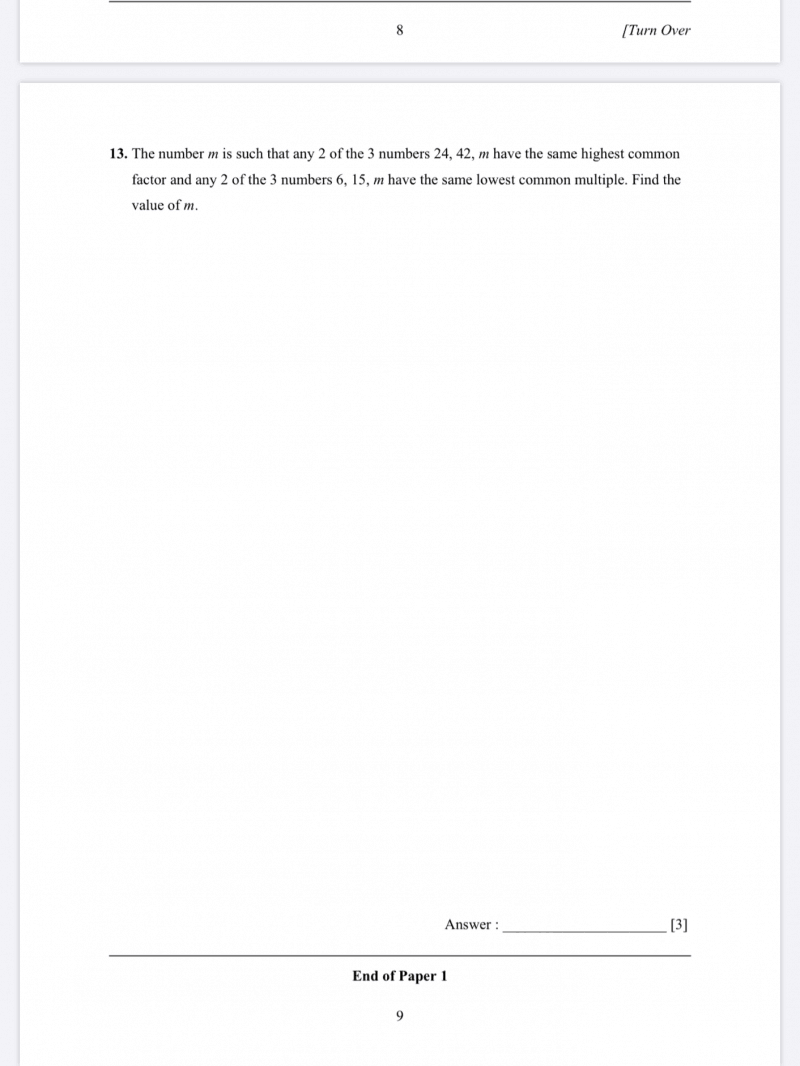# Question24 = 2 × 2 × 2 × 3
42 = 2 × 3 × 7

HCF of 24 and 42 = 2 × 3 = 6

Condition 1: The number m therefore also needs to have 6 as a factor.

6 = 2 × 3
15 = 3 × 5

LCM of 6 and 15 = 2 × 3 × 5 = 30

Condition 2: The number m therefore needs to have 30 as a multiple but should not exceed 30.

Looking at the two conditions, the only possible number is 30 itself, so m = 30.

0 Replies 1 Like ✔Accepted Answer